Computational & Technology Resources an online resource for computational,engineering & technology publications not logged in - login Civil-Comp ProceedingsISSN 1759-3433 CCP: 83PROCEEDINGS OF THE EIGHTH INTERNATIONAL CONFERENCE ON COMPUTATIONAL STRUCTURES TECHNOLOGY Edited by: B.H.V. Topping, G. Montero and R. Montenegro Paper 223Influence of Shear Deformation on the Optimal Design of a Column under Compression A. Samartin1, J.C. Mosquera1 and C. Castro21Department of Structural Mechanics, 2Department of Mathematics, E.T.S. de Ingenieros de Caminos, Canales y Puertos, Technical University of Madrid, Spain doi:10.4203/ccp.83.223 Full Bibliographic Reference for this paper A. Samartin, J.C. Mosquera, C. Castro, "Influence of Shear Deformation on the Optimal Design of a Column under Compression", in B.H.V. Topping, G. Montero, R. Montenegro, (Editors), "Proceedings of the Eighth International Conference on Computational Structures Technology", Civil-Comp Press, Stirlingshire, UK, Paper 223, 2006. doi:10.4203/ccp.83.223 Keywords: buckling, structural optimization, beam-column, eigenvalues, column stability, strongest column. Summary In this paper the optimal design of a vertical cantilever beam-column subjected to compression forces is studied. Different loads, a point load at the top section, self weight and uniform distributed vertical load, are considered. The beam-column is also assumed to be immersed in an elastic Winkler-type soil. The strongest column is defined by a structural optimization problem, namely, to find an area column-beam distribution that has the lowest volume and is able to resist the acting loading with a given safety factor. The resistance of the column is introduced by two groups of constraints: (a) admisible stresses on every column section and (b) the buckling load should be no smaller than the acting load. With this strongest column definition numerical difficulties, that are arising when classical definition is used, can be prevented. As it is known , the solution of the classical problem can be dependent on the specified minimum area. The classical strongest column is the solution of the problem of maximizing the buckling load of an Euler-Bernoulli column with a given volume. In order to solve the strongest column problem, presented in this paper, an admissible critical stress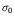is introduced. The value ofis such that both types, (a) and (b), of constrains are active, i.e. the full stress column has a buckling load equal to the acting load. Therefore, actual values of column admissible stresses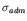are smaller thanleading to a strongest column problem called the subcritical problem with the solution given by the full stress column or column of equal strength. On the contrary, ifis greater thanthe problem is supercritical and the solution has to be obtained numerically. The solution of this problem is the main issue in this paper. The computation of the critical admissible stress implies the solution of a nonlinear equation and a Newton-Raphson technique has been applied. Typically, the first initial trial value can be the admissible stress producing the same design for a constant cross-section beam-column. In the supercritical case an iterative optimization procedure has been applied in this paper, namely, the steepest descent gradient. The full stress column has been selected as the initial feasible trial solution in this procedure. During the iterative analysis special care is taken in order to treat situations of potential multiplicity of eigenvalues. The number of iterations required to reach the solution is also shown. Beam-column have been modeled in supercritical strongest column problems by the application of the finite element (FE) method. Different FE shape functions are presented in the paper in order to test their accuracy and their efficiency. First, for Bernoulli beam-columns, classical Hermite cubic polynomials, functions corresponding to variable cross sections beams, functions related to column-beam exact homogenous behavior are introduced. Then, for Timoshenko beam-columns the used shape functions have been, in addition to the former ones, functions related to the exact solutions of Timoshenko beam and of the Timoshenko beam-column homogenous problems. Different continuity levels-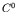,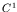and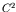- for the unknown column area distribution are also discussed in the paper, because they can obviously lead to different solutions of the strongest column problem. Also, the influence on the solution and the objective function of the quadrature formula used for column volume evaluation is commented. In an illustrative example the difference between the strongest beam column with and without shear deformation is also shown. Finally, some theoretical results related to the multiplicity order of the eigenvalues and the optimality conditions for the minimizer are given. References 1 Cox, S. J. and Oberton, M. L., "On the optimal design of columns against buckling", SIAM Journal of Mathematical Analysis, 23, 287-325, 1992. doi:10.1137/0523015 purchase the full-text of this paper (price £20) Back to top ©Civil-Comp Limited 2023 - terms & conditions